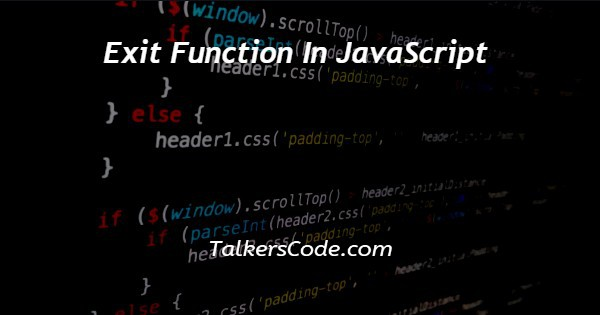# Exit Function In JavaScript

Last Updated : Jan 1, 2023In this tutorial we will show you the solution of exit function in JavaScript, here we are going to show you some example for exit a function with return statement and particular conditions.

The function call we declared with two integers to pass as parameter to function definition and there we doing some calculation with condition when it will meets condition then we exiting function with the help of return statement.

## Step By Step Guide On Exit Function In JavaScript :-

Here we defined function divi with two parameters ‘x,y’ here we checking whether dividend value it not equal to value ‘0’ then we can do division otherwise we cannot divide with divisor.

So using if condition we checked dividend ‘y’ value and doing division operation.

When we meets defined condition or proceeds operation there we used return statement for exit the function divi() then printed on webpage.

```<!DOCTYPE html>
<html>
<title>EXIT FUNCTION</title>
<body>
<script>
function divi(x,y){
if(y==0){
return "invalid y";
}
else return x/y;
}
console.log("x=9","y=3","9/3 :",divi(9,3));
console.log("x=10","y=0","10/0 :",divi(10,0));
</script>
</body>
</html>```
1. <!DOCTYPE html> tag which is instruct the web browser about what version of HTML file written in and it’s not have any ending tag.
2. The<html> tag is used to indicate the beginning of HTML document.
3. As above shown <head> tag is contain information about webpage and external file links are declared here. <title> tag is used for set the webpage title.
4. Both <head> and <title> tags having their pair end tag, so we need to close the ending tags respectively. If you’re not closed anyone of ending tag properly that is also affect the webpage result.
5. <body> tag is beginning of main coding part because it contain coding of entire website blocks and elements described here.
6. In script we defined divi() function with two parameters ‘x,y’ there we checking whether ‘y’ value is ‘0’ or not.
7. Because here we doing division operation then our dividend must be a non-zero value otherwise we can’t do the division operation.
8. If condition true means ‘y’ value must be ‘0’ then we returning message ‘invalid y’ to function declaration because we declared with two integer values at print statement of ‘console.log()’.
9. If false means then we doing division operation successfully and returned result to print state. We declared print statement two times with different parameters of integers for user clarification.
10. Which is shows you two possible outputs and printed result on console panel.
11. Both </body>,</html> tags closed respectively. </body> tag indicates the end of body, Then </html> tag indicates the end of HTML document.

## Conclusion :-

In conclusion now we are able to know how to exit in function using JavaScript.

When we executes program on browser we can’t see the result because we printed on console panel so we have to use shortcut ‘ctrl+shift+j’ then console panel will open at right hand side with result messages of ‘x=9 y=3 9/3 : 3, x=10 y=0 10/0 : invalid y’.

If your need to do this with some other integers you can simply modify that we can see result without error.

I hope this tutorial on exit function in JavaScript helps you and the steps and method mentioned above are easy to follow and implement.